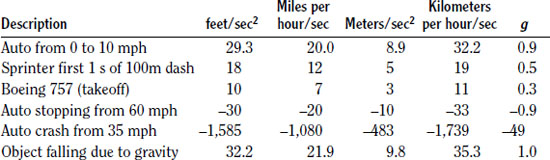NextPrevious

# What is acceleration?

Speed and velocity are seldom constant. Usually they vary, and acceleration is a description of how that variation occurs. Acceleration is called the time rate of change of velocity. That is, it is the change in velocity divided by the time over which the change occurs. For example, a car might accelerate from 0 to 60 miles per hour. A sports car might do this in five seconds, while an economy car might require nine seconds. Which car has the larger acceleration? Both have the same change in speed, but the sports car requires less time to make the change, so it has the larger acceleration.

Like velocity, acceleration is a vector. That is, it includes both magnitude and direction. In speeding up, in which the change in velocity is positive, acceleration is a positive quantity. If the object is slowing down then the acceleration is negative. If, however, the object is going in the negative direction—for example, a car going in reverse—then speeding up is a negative quantity because the final velocity is more negative than the initial velocity.

Physicists do not use the term deceleration. Whether positive or negative, speeding up or slowing down, the term acceleration is always used.

The table below shows some typical accelerations in a variety of units. The rightmost column, labeled “g,” compares the acceleration to that of an object falling in Earth’s gravitational field. This ratio is frequently used in both everyday and scientific writing.Close

This is a web preview of the "The Handy Physics Answer Book" app. Many features only work on your mobile device. If you like what you see, we hope you will consider buying. Get the App